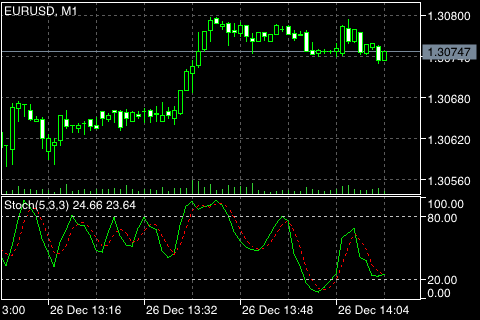# Stochastic Oscillator

The Stochastic Oscillator Technical Indicator compares where a securitys price closed relative to its price range over a given time period. The Stochastic Oscillator is displayed as two lines. The main line is called %K. The second line called %D is a Moving Average of %K. The %K line is usually displayed as a solid line and the %D line is usually displayed as a dotted line. There are several ways to interpret a Stochastic Oscillator. Three popular methods include:

• Buy when the Oscillator (either %K or %D) falls below a specific level (for example, 20) and then rises above that level. Sell when the Oscillator rises above a specific level (for example, 80) and then falls below that level.
• Buy when the %K line rises above %D. Sell when %K falls below %D.
• Look for divergences. For instance: where prices are making a series of new highs and the Stochastic Oscillator is failing to surpass its previous highs.## Calculation

Four variables are used for the calculation of the Stochastic Oscillator:

• %K periods. This is the number of time periods used in the stochastic calculation.
• %K Slowing Periods. This value controls the internal smoothing of %K. A value of 1 is considered a fast stochastic; a value of 3 is considered slow.
• %D periods. This is the number of time periods used when calculating a moving average of %K.
• %D method. The method (i.e., Exponential, Simple, Smoothed, or Weighted) that is used to calculate %D.

The formula for %K is:

%K = (CLOSE - MIN (LOW (%K))) / (MAX (HIGH (%K)) - MIN (LOW (%K))) * 100

Where:

CLOSE – todays closing price;
MIN (LOW (%K)) – the lowest low for the %K periods;
MAX (HIGH (%K)) – the highest high for the %K periods.

The %D moving average is calculated according to the formula:

%D = SMA (%K, N)

Where:

N – smoothing period;
SMA – Simple Moving Average.